# GCSE Calculator Questions 2 (2013/Higher Tier)

This worksheet contains a sample of practice questions based on the 2013 GCSE Higher Level syllabus. Calculators may be used.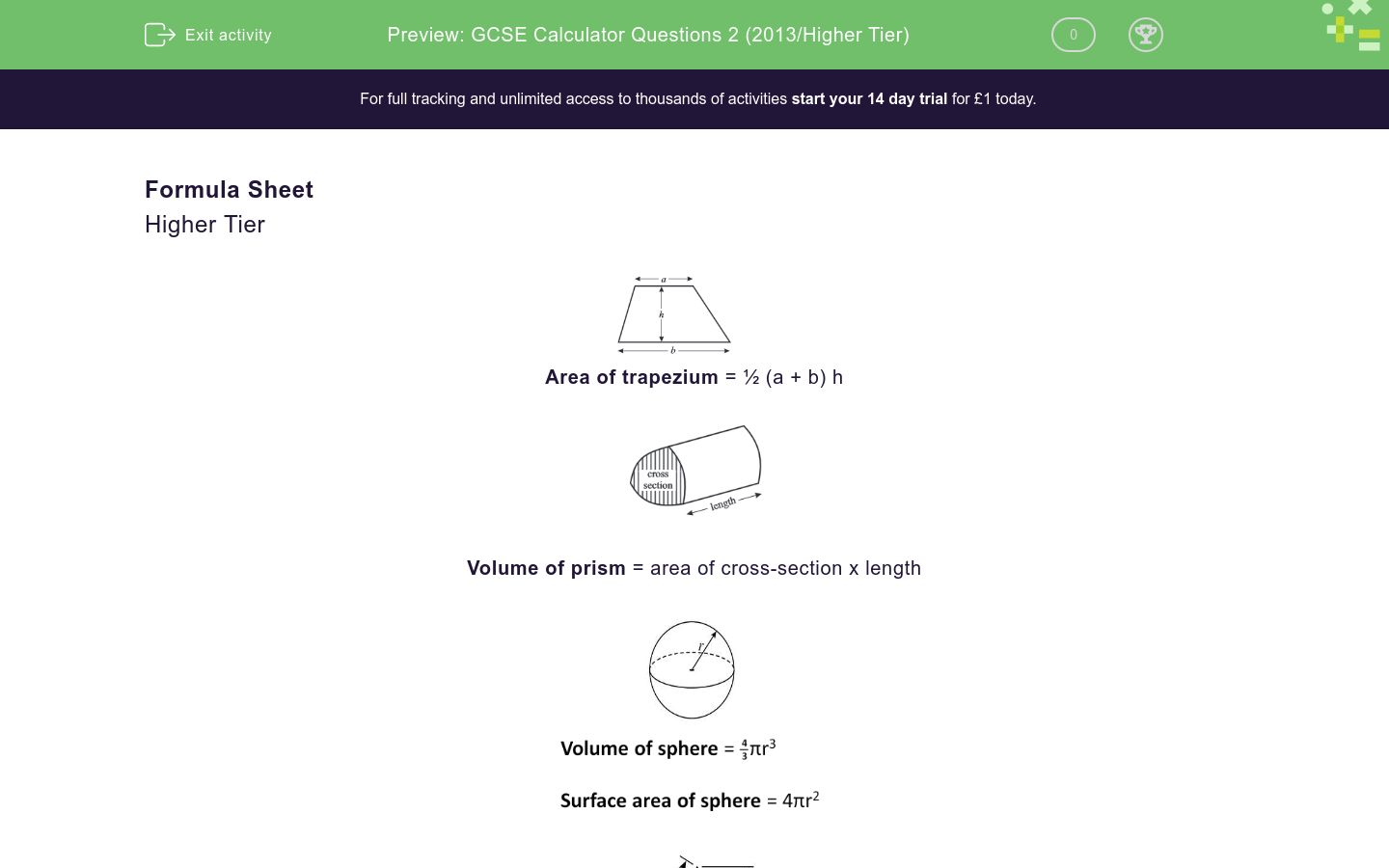Key stage:  KS 4

Curriculum topic:  GCSE Practice Papers

Curriculum subtopic:  Selection of Topics for Calculator Practice

Difficulty level:### QUESTION 1 of 10

Formula Sheet

Higher TierArea of trapezium = ½ (a + b) hVolume of prism = area of cross-section x length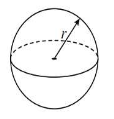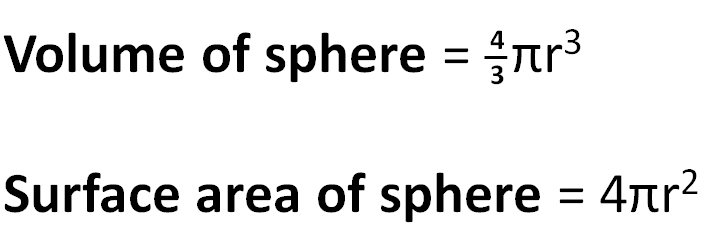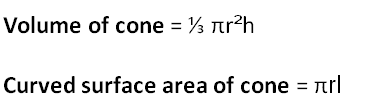In any triangle ABC:

Area of triangle = ½ab sinC

Sine rule

 a = b = c sin A sin B sin C

Cosine rule

a2 = b2 + c2 - 2bc cos A

The quadratic equation:

The solutions of ax2 + bx + c = 0, where a ≠ 0, are given byBen thinks of a number.

He multiplies it by 3.

He then adds 4.

He then squares the result.

His final answer is 432.64.

What number did he first think of?

What is the smallest integer that satisfies this inequality:

5x - 7 > 12 - x

Fatima bakes a small round cake of diameter 11 cm.She wishes to put a ribbon around the circumference of the cake.

Calculate the least length of ribbon that she can use.

(Just write the number to 3 sig. figs)

Write the following fraction as a decimal:

 9 40

The diagram shows a circle centre C.

Work out the value of angle a.

(Just write the number)Calculate the value of the following to 3 decimal places:

 √7.36 - 5.14 3.98

The diagram below shows a  triangle PQR.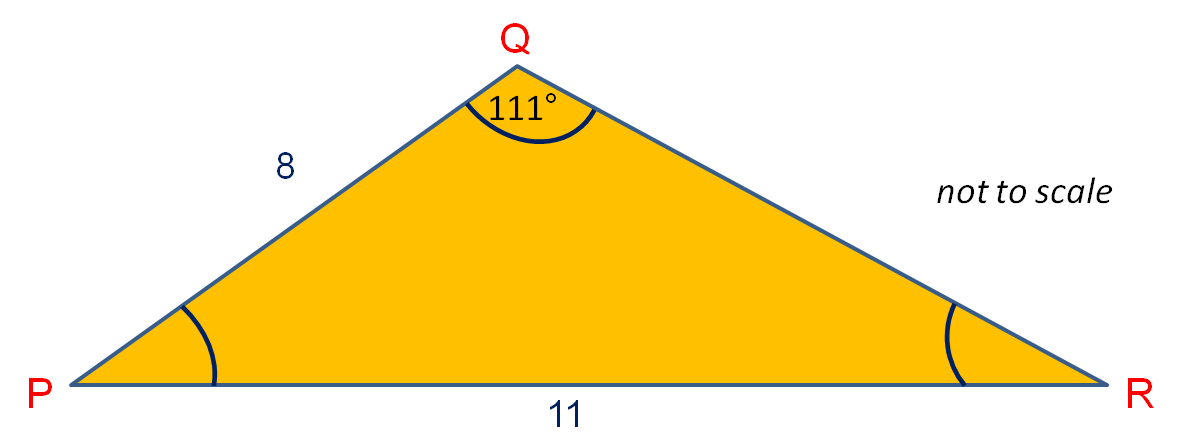Use the sine rule to find ∠QPR to 1 dp.

(Just write the number)

Use the quadratic formula to solve for x, giving your answers to 3 sig. figs.

3x2 = 19x - 2

x = 6.23

x = 0.407

x = 0.623

x = 0.107

After a 12% reduction in price, a bicycle costs £109.12 in the sale.

How much did it cost in pounds before the sale?

Simplify the following and select the correct answer:

 a + 4 - a - 3 4 8

Answers

 1 a + 11 2. 9a + 8 3. 2a + 18 8 32 12

1.

2.

3.

The diagram shows two fair spinners.Both spinners are spun and the sum of the scores is found.

Calculate the probability that the sum of the scores is 10 or more.

(Give your answer as a reduced fraction in the form a/b)

ABCDEFGH is a cuboid.

Select the coordinates of the midpoint of FG.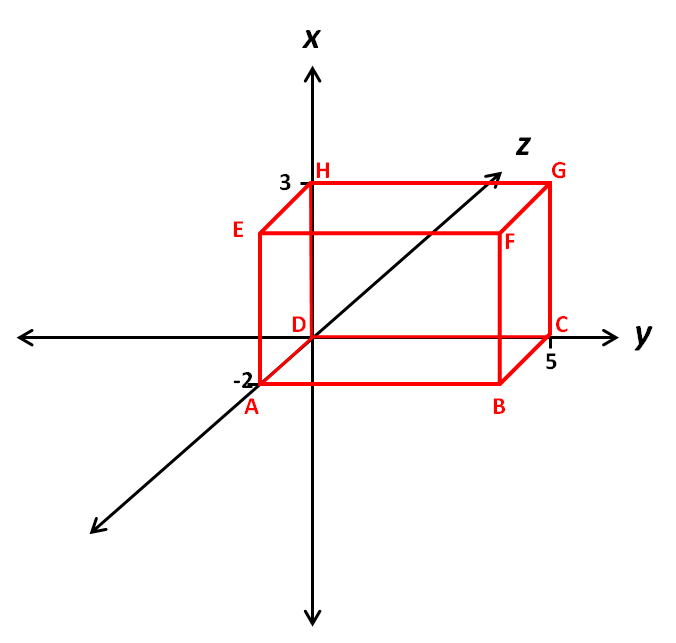(5, -1, 3)

(3, 5, -1)

(5½, -1, 3)

(5, 1, 3)

(3, 5, 1)

(-½, 3, 5)

ABCDEFGH is a cuboid.

Calculate the length BH to 3 sig. figs.Here is a formula.

s = ut + ½at2

Calculate s when u = 6.2 x 102, t = 4.5 and a = 9.8

(Give your answer in standard form to 3 sig. figs.)

2.89 × 106

28.9 × 102

2.88 × 103

2.89 × 103

2889 × 103

Eight men can dig a trench in 3 hours.

How long will it take five men to dig a similar trench of the same size?

4 hours 8 mins

13 hours 20 mins

4 hours 48 mins

4 hours 80 mins

5 hours 20 mins

The pilot of a plane wishes to fly due North but there is an 80 mph Easterly wind (i.e. from the East.  The plane's airspeed is 200 mph.  At what bearing must the pilot fly the plane in order to head due North?

(Just write the three figure number)Solve the equation:

(2x + 1)2 = (x + 5)2

x = -4, -2

x = -4, 2

x = 8, -1

x = 4, 2

x = 4, -2

A cyclist travels 25 kilometres at a speed of 20 km/h and then another 80 km at a speed of 25 km/h.

Calculate the cyclist's average speed for the whole journey in km/h to 1 decimal place.

(Just write the number)

The marks of 15 children in a maths test are represented on a Stem and Leaf Diagram as shown below.

Work out the interquartile range.

 Stem Leaf 0 7   9 1 1   4   4   7 2 0   3   3   3   9 3 0   4   4 4 4

The diagram shows a solid prism made from a metal with density of 5.8 grams per cm3.(measurements in cm, but not drawn accurately)

Calculate the mass of the prism in kilograms.

(Just write the number)

Jack's mass increases from 45.6 kg to 52.4 kg.

Calculate the percentage increase in Jack's mass.

(Just write the number to 3 significant figures)

Calculate the side length x to 3 sig. figs.(Diagram not drawn accurately)

Charlie is experimenting with seedlings and measures the height of his seedling plants after two weeks.  The results are recorded in this frequency table.

Work out the Frequency Density for the 25 ≤ h < 30 class interval.

(Just write the number)In △ABC above,

c = 14 cm

a = 9.5 cm

∠ABC = 80.5º

Calculate its area in cm2 to 3 s.f.

(Just write the number)

Rearrange the following formula to make a the subject.

s = ut + ½at2

a = (s - ½at²)/t

a = 2(s - ut)/t²

a = sut²

a = ½s - ut²

• Question 1

Ben thinks of a number.

He multiplies it by 3.

He then adds 4.

He then squares the result.

His final answer is 432.64.

What number did he first think of?

CORRECT ANSWER
5.6
EDDIE SAYS
We work backwards, carrying out the inverse of each operation. √432.64 = 20.8
20.8 - 4 = 16.8
16.8 ÷ 3 = 5.6
• Question 2

What is the smallest integer that satisfies this inequality:

5x - 7 > 12 - x

CORRECT ANSWER
4
EDDIE SAYS
5x - 7 > 12 - x
6x - 7 > 12
6x > 19
x > 19/6 = 3.166666...
Smallest integer greater than 3.16... is therefore 4
• Question 3

Fatima bakes a small round cake of diameter 11 cm.She wishes to put a ribbon around the circumference of the cake.

Calculate the least length of ribbon that she can use.

(Just write the number to 3 sig. figs)

CORRECT ANSWER
34.6
EDDIE SAYS
Diameter d = 11cm
Circumference = π × d = π × 11 = 34.5575 cm
• Question 4

Write the following fraction as a decimal:

 9 40

CORRECT ANSWER
0.225
EDDIE SAYS
9 ÷ 40 = 0.225
• Question 5

The diagram shows a circle centre C.

Work out the value of angle a.

(Just write the number)CORRECT ANSWER
42
EDDIE SAYS
Triangle ABC is isosceles because AC and BC are both radii of the circle.
∠ACB = 180° - 2 × 48° = 84°
Angle at circumference is twice angle at centre.
Angle a = ½ of 84° = 42°
• Question 6

Calculate the value of the following to 3 decimal places:

 √7.36 - 5.14 3.98
CORRECT ANSWER
-0.610
EDDIE SAYS
On the calculator, type:
(√7.36 - 5.14)÷ 3.98 =
-0.609816082 rounds to -0.610 to 3 decimal places.
• Question 7

The diagram below shows a  triangle PQR.Use the sine rule to find ∠QPR to 1 dp.

(Just write the number)

CORRECT ANSWER
26.2
EDDIE SAYS
 11 = 8 sin 111° sin ∠QRP

∠QPR = 180° - 111° - ∠QRP
• Question 8

Use the quadratic formula to solve for x, giving your answers to 3 sig. figs.

3x2 = 19x - 2

CORRECT ANSWER
x = 6.23
x = 0.107
EDDIE SAYS
Rearrange to read 3x² - 19x + 2 = 0
Use the quadratic formula, substituting a = 3, b = -19 and c = 2
• Question 9

After a 12% reduction in price, a bicycle costs £109.12 in the sale.

How much did it cost in pounds before the sale?

CORRECT ANSWER
£124
£ 124
EDDIE SAYS
When we reduce something by 12%, we multiply it by 0.88
109.12 ÷ 0.88 = 124
• Question 10

Simplify the following and select the correct answer:

 a + 4 - a - 3 4 8

Answers

 1 a + 11 2. 9a + 8 3. 2a + 18 8 32 12

CORRECT ANSWER
1.
EDDIE SAYS
Multiply top and bottom of first fraction by 2 to get (2a + 8)/8
Then combine the tops to get 2a + 8 - a + 3 = a + 11
• Question 11

The diagram shows two fair spinners.Both spinners are spun and the sum of the scores is found.

Calculate the probability that the sum of the scores is 10 or more.

(Give your answer as a reduced fraction in the form a/b)

CORRECT ANSWER
3/16
EDDIE SAYS
There are 4 × 8 = 32 outcomes.
Of these 2+8, 3+7, 3+8, 4+6, 4+7, 4+8 are the only six outcomes that give a sum of 10 or more.
Probability = 6/32 = 3/16.
• Question 12

ABCDEFGH is a cuboid.

Select the coordinates of the midpoint of FG.CORRECT ANSWER
(3, 5, -1)
EDDIE SAYS
F is at (3, 5, -2) and G is at (3, 5, 0)
• Question 13

ABCDEFGH is a cuboid.

Calculate the length BH to 3 sig. figs.CORRECT ANSWER
6.16
EDDIE SAYS
By Pythagoras' Theorem, BH² = 5² + 2² + 3² since the dimension of the cuboid are 5 by 2 by 3.
BH² = 25 + 4 + 9 = 38
BH = √38 = 6.16 (3 sf)
• Question 14

Here is a formula.

s = ut + ½at2

Calculate s when u = 6.2 x 102, t = 4.5 and a = 9.8

(Give your answer in standard form to 3 sig. figs.)

CORRECT ANSWER
2.89 × 103
EDDIE SAYS
s = 6.2 × 102 × 4.5 + 0.5 × 9.8 × 4.52 = 2889.225
• Question 15

Eight men can dig a trench in 3 hours.

How long will it take five men to dig a similar trench of the same size?

CORRECT ANSWER
4 hours 48 mins
EDDIE SAYS
8 men take 3 hours.
1 man would take 8 × 3 = 24 hours.
5 men would take 24 ÷ 5 = 4.8 hours = 4 hours 48 mins.
Remember that 0.8 hour = 0.8 × 60 = 48 mins.
• Question 16

The pilot of a plane wishes to fly due North but there is an 80 mph Easterly wind (i.e. from the East.  The plane's airspeed is 200 mph.  At what bearing must the pilot fly the plane in order to head due North?

(Just write the three figure number)CORRECT ANSWER
024
EDDIE SAYS
sin θ° = 80/200 = 0.4
θ° = sin-10.4 = 23.6
Bearing has three digits
• Question 17

Solve the equation:

(2x + 1)2 = (x + 5)2

CORRECT ANSWER
x = 4, -2
EDDIE SAYS
(2x + 1)(2x + 1) = (x + 5)(x + 5)
4x² + 4x + 1 = x² + 10x + 25
3x² -6x - 24 = 0
x² -2x - 8 = 0
(x - 4)(x + 2) = 0
x = 4, -2
• Question 18

A cyclist travels 25 kilometres at a speed of 20 km/h and then another 80 km at a speed of 25 km/h.

Calculate the cyclist's average speed for the whole journey in km/h to 1 decimal place.

(Just write the number)

CORRECT ANSWER
23.6
EDDIE SAYS
Total time taken = 25/20 + 80/25 = 4.45 hours.
Total distance cycled = 25 + 80 = 105 km.
Average speed = 105/4.45 =23.6 km/h
• Question 19

The marks of 15 children in a maths test are represented on a Stem and Leaf Diagram as shown below.

Work out the interquartile range.

 Stem Leaf 0 7   9 1 1   4   4   7 2 0   3   3   3   9 3 0   4   4 4 4

CORRECT ANSWER
6
EDDIE SAYS
The lower quartile is the median of the lowest 7 numbers, which is 14.
The upper quartile is the median of the highest 7 numbers, which is 30.
The interquartile range is 30 - 14 = 6.
• Question 20

The diagram shows a solid prism made from a metal with density of 5.8 grams per cm3.(measurements in cm, but not drawn accurately)

Calculate the mass of the prism in kilograms.

(Just write the number)

CORRECT ANSWER
1.4094
EDDIE SAYS
Volume of prism = (3 × 5 + 6 × 2) × 9 = 27 × 9 = 243 cm³
Mass = 243 × 5.8 = 1409.4 g = 1.4094 kg
• Question 21

Jack's mass increases from 45.6 kg to 52.4 kg.

Calculate the percentage increase in Jack's mass.

(Just write the number to 3 significant figures)

CORRECT ANSWER
14.9
EDDIE SAYS
Actual increase = 52.4 - 45.6 = 6.8
Percentage increase = 6.8/45.6 × 100 = 14.9%
• Question 22

Calculate the side length x to 3 sig. figs.(Diagram not drawn accurately)

CORRECT ANSWER
8.90
EDDIE SAYS
x = 6 ÷ tan34°
• Question 23

Charlie is experimenting with seedlings and measures the height of his seedling plants after two weeks.  The results are recorded in this frequency table.

Work out the Frequency Density for the 25 ≤ h < 30 class interval.

(Just write the number)CORRECT ANSWER
2.2
EDDIE SAYS
Class width = 30 - 25 = 5
Frequency density = Frequency ÷ Class Width = 11 ÷ 5 = 2.2
• Question 24In △ABC above,

c = 14 cm

a = 9.5 cm

∠ABC = 80.5º

Calculate its area in cm2 to 3 s.f.

(Just write the number)

CORRECT ANSWER
65.6
EDDIE SAYS
Area = ½ × 9.5 × 14 × sin80.5° = 65.58799...cm²
• Question 25

Rearrange the following formula to make a the subject.

s = ut + ½at2

CORRECT ANSWER
a = 2(s - ut)/t²
EDDIE SAYS
Subtract ut from both sides, double both sides and divide by t²
---- OR ----

Sign up for a £1 trial so you can track and measure your child's progress on this activity.

### What is EdPlace?

We're your National Curriculum aligned online education content provider helping each child succeed in English, maths and science from year 1 to GCSE. With an EdPlace account you’ll be able to track and measure progress, helping each child achieve their best. We build confidence and attainment by personalising each child’s learning at a level that suits them.

Get startedStart your £1 trial today.
Subscribe from £10/month.